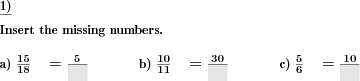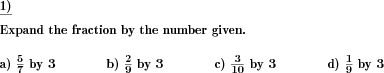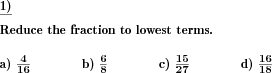Custom math worksheets at your fingertips# Details for problem "Missing elements in unlike fractions "

Quickname: 6652

Elementary School, Primary School, Junior High School, Middle School, High School.

## Summary

In unlike fractions of the same value, numerators or denominators have to be filled in.

## Example## Description

For every problem, two fractions representing the same value are given. In the second fraction, the numerator or denominator is missing as has to be filled in.

The number of problems is selectable. It can be chosen what element(s) may be missing in the second fraction, the numerator only, the denominator only, or a random element.

As both fractions represent the same quotient, the second fraction will be a reduced or expanded variant of the first one. It is selectable whether is will reduced only, expanded only, or a mix of both.

Download free printable worksheets for this math problem here. The worksheet contains the problems only, the solution sheet includes the answers. Just click on the respective link.

•Worksheet 1Solution sheet with answers
•Worksheet 2Solution sheet with answers
•Worksheet 3Solution sheet with answers

If you can not see the solution sheets for download, they may be filtered out by an ad blocker that you may have installed. If this is the case, please allow ads for this page and reload the page. The solution sheets will then reappear.

• Do these sample worksheets do not really fit?
• Do you need more math worksheets, with a different level of difficulty?
• Would you like to combine different problems on a worksheet and adjust them to your needs?
• As a teacher, you can put together your own worksheets using the automatically generated math problems provided.
With a free initial credit, you can start creating your own math worksheets in a few minutes.

You can try it for free! Register here, to create custom worksheets now!

## Customization options for this problem

Parameter
Possible values
Number of problems
1, 2, 3, 4, 5, 6, 7, 8, 9, 10
Number range
20, 30, 40, 50, 100, 200, 500, 1000, 10000, 100000
Second fraction is
Mixed, Expanded only, Reduced only
Numbers missing in
Mixed, Numerator, Denominator

## dw-Math worksheet templates that do contain this problem

Name
Title
Description
Reducing and expanding fractions
Expansion and reduction of fractions, and converting fractions to like quantities.

## Other types of problems that appear on worksheets with this problem:

Relevance
Name
Description
Quickname
Example
****
Expand fractions by given number
Fractions are to be expanded by a given number****
Convert fractions to like fractions
Convert multiple fractions to like quantities****
Reduce fractions
A fraction has to be reduced to lowest terms.